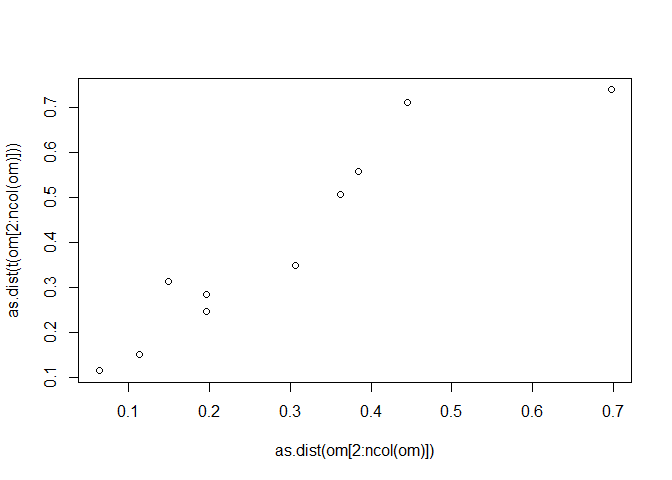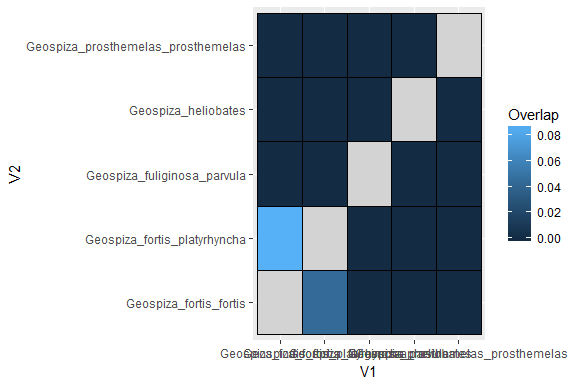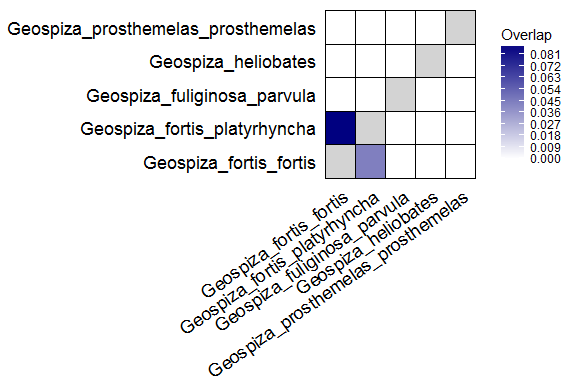# Quantification of size and overlap of n-dimensional hypervolumes in R: dynRB tutorial

## Abstract

This tutorial demonstrates the use of the R package dynamic range boxes dynRB to quantify the size and overlap of n-dimensional hypervolumes. It provides information on formatting data for the use in dynRB, introduces the functions implemented in the package, explains the outputs, gives examples on how to use the output for follow-up analyses, and finally shows how to visualize the results.

## Required packages

The quantification of size and overlap of n-dimensional hypervolumes requires the package dynRB (a detailed description of the method can be found in Junker et al. 2016). Additionally, the packages ggplot2, reshape2, vegan, and RColorBrewer are required for follow-up analyses demonstrated in this tutorial.

library(dynRB)
library(ggplot2)
library(reshape2)
library(vegan)
library(RColorBrewer)

## Formatting data for use in dynRB

The grouping variable (e.g. species) is required to be in the first column of the data table, followed by two or more columns containing continuous variables representing the different dimensions of the n-dimensional hypervolume. Thus, each row contains the measurements of one unit of interest (e.g. an individual of a species) in column 2 and the following columns as well as the grouping variable for this unit (first column). dynRB does not require complete measurements of all continuous variables in each row; missing values (i.e. NA) are omitted during the analysis. The package dynRB provides an example data set “finch”“, which serves as a reference. The”finch" data set includes morphological measurements of Darwin finches Geospiza sp., which originates from Snodgrass and Heller (1904) and was extracted from the R package hypervolume (Blonder et al. 2014). It comprises quantitative measurements of nine traits characterizing five (sub-) species of finches, each trait was measured at least in 10 individuals per species (see also Junker et al. 2016).

data(finch)
head(finch)[,1:5]
##               Species BodyL WingL TailL BeakW
## 1 Geospiza_heliobates   123  72.0  48.5   6.5
## 2 Geospiza_heliobates   126  70.0  48.5   7.0
## 3 Geospiza_heliobates   133  71.5  45.0   6.5
## 4 Geospiza_heliobates   127  69.0  39.0   6.5
## 5 Geospiza_heliobates   122  71.0  42.0   6.7
## 6 Geospiza_heliobates   128  72.0  43.0   6.5

The finch data set contains measurements of nine traits of 146 bird individuals belonging to five species. For a weighted analysis putting more weight on some individuals than on others, these individuals need to appear in two or more rows of the data table (compare to Kuppler et al. 2017; Junker & Larue-Kontic 2018). For instance, in Junker & Larue-Kontic (2018) we quantified the size and overlap of the trait spaces occupied by plant communities with plant traits as dimensions. In order to consider the abundances of the plant species within each of the communities, trait-data of each species was inserted in a number of rows proportional to the abundance of the species in the community. Let us assume species A has an abundance of 10 individuals and species B 20 individuals in the same community; in this case trait-data of species A would appear in 10 rows and those of species B in 20 rows.

## Quantification of size and overlap of n-dimensional hypervolumes

The main function of the package dynRB “dynRB_VPa” quantifies the size and the overlap of the n-dimensional hypervolumes of two or more objects (e.g. the five finch species in the example data).

r <- dynRB_VPa(finch)

Dynamic range boxes first calculates the size (vol) and overlap (port) individually for each dimension and then aggregates the dimensions in order to quantify the size and overlap of the n-dimensional hypervolumes. Both size and overlap are bounded between 0 and 1, with values near 0 indicating a small size and overlap and near 1 a large size and overlap. Three different aggregation methods are provided:

• Product: size and overlap of each of the dimensions are multiplied. Hypervolumes become zero if size or overlap is zero in one of the dimensions. The size and overlap are dependent on the number of dimensions and thus, size and overlap of hypervolumes based on different number of dimensions are not comparable.

• Mean: the mean size and overlap of the dimensions. Hypervolumes do not become zero if size or overlap is zero in one of the dimensions. The size and overlap are not biased by the number of dimensions and thus comparable between hypervolumes with different numbers of dimensions.

• Gmean: the geometric mean size and overlap of the dimensions. Hypervolumes become zero if size or overlap is zero in one of the dimensions. The size and overlap are not biased by the number of dimensions and thus comparable between hypervolumes with different numbers of dimensions.

res <- r$result res[1:6, 1:5] ## V1 V2 ## 1 Geospiza_fortis_fortis Geospiza_fortis_fortis ## 2 Geospiza_fortis_platyrhyncha Geospiza_fortis_fortis ## 3 Geospiza_fuliginosa_parvula Geospiza_fortis_fortis ## 4 Geospiza_heliobates Geospiza_fortis_fortis ## 5 Geospiza_prosthemelas_prosthemelas Geospiza_fortis_fortis ## 6 Geospiza_fortis_fortis Geospiza_fortis_platyrhyncha ## port_prod port_mean port_gmean ## 1 1.00000000 1.0000000 1.0000000 ## 2 0.04410650 0.6968772 0.6792476 ## 3 0.00000000 0.1968984 0.0000000 ## 4 0.00000000 0.4453380 0.0000000 ## 5 0.00000000 0.1497419 0.0000000 ## 6 0.08855663 0.7387798 0.7391182 Columns V1 and V2 denote the species pair considered. Columns “port_prod”, “port_mean”, and “port_gmean” contain the overlap of the hypervolumes of the species given in the first two columns (considering the afore-mentioned aggregation methods). Thus, each of the three columns show the overlap between V1 and V2 expressed as the proportion of the hypervolume of V2 that is covered by the hypervolume of V1 (port(V1, V2)). Since the overlap is expressed as proportion it is dependent on the size of the hypervolumes and thus, port(V1, V2) is not the same as port(V2, V1) since overlaps are, by construction, asymmetric. res[1:6, c(1:2, 6:11)] ## V1 V2 ## 1 Geospiza_fortis_fortis Geospiza_fortis_fortis ## 2 Geospiza_fortis_platyrhyncha Geospiza_fortis_fortis ## 3 Geospiza_fuliginosa_parvula Geospiza_fortis_fortis ## 4 Geospiza_heliobates Geospiza_fortis_fortis ## 5 Geospiza_prosthemelas_prosthemelas Geospiza_fortis_fortis ## 6 Geospiza_fortis_fortis Geospiza_fortis_platyrhyncha ## vol_V1_prod vol_V1_mean vol_V1_gmean vol_V2_prod vol_V2_mean ## 1 1.068481e-03 0.8198984 0.8099190 0.001068481 0.8198984 ## 2 5.176950e-04 0.7928641 0.7804499 0.001068481 0.8198984 ## 3 5.199396e-05 0.6698550 0.6332720 0.001068481 0.8198984 ## 4 9.560829e-06 0.6416451 0.5775970 0.001068481 0.8198984 ## 5 7.759617e-07 0.5283642 0.4651936 0.001068481 0.8198984 ## 6 1.068481e-03 0.8198984 0.8099190 0.000517695 0.7928641 ## vol_V2_gmean ## 1 0.8099190 ## 2 0.8099190 ## 3 0.8099190 ## 4 0.8099190 ## 5 0.8099190 ## 6 0.7804499 Colums “vol_V1_prod”, “vol_V1_mean”, and “vol_V1_gmean” give the size vol(V1) of the hypervolume of species V1 expressed by the different aggregation methods. Colums “vol_V2_prod”, “vol_V2_mean”, and “vol_V2_gmean” contain the size vol(V2) of the hypervolume of species V2. ## Quantification of overlaps per dimension The function of the package dynRB “dynRB_Pn” quantifies the overlap per dimension of the n-dimensional hypervolumes of two or more objects (e.g. the five finches in the example data). r <- dynRB_Pn(finch) head(r$result)
##                                   V1                           V2
## 1             Geospiza_fortis_fortis       Geospiza_fortis_fortis
## 2       Geospiza_fortis_platyrhyncha       Geospiza_fortis_fortis
## 3        Geospiza_fuliginosa_parvula       Geospiza_fortis_fortis
## 4                Geospiza_heliobates       Geospiza_fortis_fortis
## 5 Geospiza_prosthemelas_prosthemelas       Geospiza_fortis_fortis
## 6             Geospiza_fortis_fortis Geospiza_fortis_platyrhyncha
##        BodyL      WingL     TailL     BeakW     BeakH     LBeakL
## 1 1.00000000 1.00000000 1.0000000 1.0000000 1.0000000 1.00000000
## 2 0.15453928 0.18277541 0.3425038 0.3332417 0.3365428 0.42295060
## 3 0.09170653 0.01256925 0.3517214 0.0000000 0.0000000 0.00000000
## 4 0.70330170 0.45452466 0.4339771 0.0000000 0.0000000 0.07690136
## 5 0.01640114 0.00000000 0.1921549 0.0000000 0.0000000 0.00000000
## 6 0.17338308 0.23404235 0.5712031 0.1904819 0.2457569 0.35876038
##        UBeakL     N.UBkL    TarsusL
## 1 1.000000000 1.00000000 1.00000000
## 2 0.465146882 0.36397853 0.17044059
## 3 0.000000000 0.00000000 0.01834062
## 4 0.004597931 0.07327674 0.25627463
## 5 0.000000000 0.00000000 0.12326127
## 6 0.482662774 0.30233807 0.52381250

Columns V1 and V2 denote the species pair considered. The following columns give the overlap port(V1, V2) for each of the dimensions contained in the data set.

## Quantification of sizes per dimension

The function of the package dynRB “dynRB_Vn” quantifies the size per dimension of the n-dimensional hypervolume for each of the objects (e.g. the five finches in the example data).

r <- dynRB_Vn(finch)
head(r$result) ## V1 BodyL WingL TailL ## 1 Geospiza_fortis_fortis 0.4647516 0.4854382 0.6397608 ## 2 Geospiza_fortis_platyrhyncha 0.5025632 0.2950539 0.4697389 ## 3 Geospiza_fuliginosa_parvula 0.2977510 0.2727177 0.4158340 ## 4 Geospiza_heliobates 0.3996047 0.2244853 0.4796407 ## 5 Geospiza_prosthemelas_prosthemelas 0.2335282 0.1024436 0.3945226 ## BeakW BeakH LBeakL UBeakL N.UBkL TarsusL ## 1 0.2540664 0.35182958 0.40009301 0.28362837 0.38422774 0.3494316 ## 2 0.6023780 0.46275581 0.35257509 0.41874272 0.35080020 0.2235962 ## 3 0.1238108 0.09686040 0.12803845 0.26326448 0.16692633 0.2501269 ## 4 0.1088183 0.19777844 0.10500633 0.03590313 0.22488576 0.3278321 ## 5 0.1230323 0.08207679 0.07150991 0.10152492 0.03588937 0.1710150 Column V1 denotes the species considered. The following columns give the size vol(V1) for each of the dimensions contained in the data set. ## Transformation of the output (to matrix format) Many follow-up analyses may require another data format as the one provided by dynRB. Often matrices are required with the groups as row and column names and the pairwise overlap as entries in the cells. r <- dynRB_VPa(finch) # aggregation method product om1 <- reshape(r$result[,1:3], direction="wide", idvar="V1", timevar="V2")
#aggregation method mean
om2 <- reshape(r$result[,c(1,2,4)], direction="wide", idvar="V1", timevar="V2") #aggregation method gmean om3 <- reshape(r$result[,c(1,2,5)], direction="wide", idvar="V1", timevar="V2") 
om1
##                                   V1 port_prod.Geospiza_fortis_fortis
## 1             Geospiza_fortis_fortis                        1.0000000
## 2       Geospiza_fortis_platyrhyncha                        0.0441065
## 3        Geospiza_fuliginosa_parvula                        0.0000000
## 4                Geospiza_heliobates                        0.0000000
## 5 Geospiza_prosthemelas_prosthemelas                        0.0000000
##   port_prod.Geospiza_fortis_platyrhyncha
## 1                             0.08855663
## 2                             1.00000000
## 3                             0.00000000
## 4                             0.00000000
## 5                             0.00000000
##   port_prod.Geospiza_fuliginosa_parvula port_prod.Geospiza_heliobates
## 1                                     0                             0
## 2                                     0                             0
## 3                                     1                             0
## 4                                     0                             1
## 5                                     0                             0
##   port_prod.Geospiza_prosthemelas_prosthemelas
## 1                                            0
## 2                                            0
## 3                                            0
## 4                                            0
## 5                                            1

Each element in this matrix is identified by its row number, which corresponds to one particular group, and its column number, which corresponds to another group. For example, om[1,3] refers to the entry in the first row and third column of the table. Its interpretation is as follows. It is the proportion of the hypervolume of the third column group (Geospiza fortis platyrhyncha) that is overlapped by the hypervolume of the first row group (Geospiza fortis fortis), i.e. port(Geospiza fortis platyrhyncha, Geospiza fortis fortis).

## Evaluation of the asymmetry in overlaps

One example to analyze the output of dynamic range boxes is to evaluate the asymmetry of overlaps using a Mantel test. Therefore, the lower half of the matrix generated from the output of dynRB needs to be correlated with the upper half of the same matrix.

r <- dynRB_VPa(finch)
#aggregation method mean
om <- reshape(r$result[,c(1,2,4)], direction="wide", idvar="V1", timevar="V2") mantel(as.dist(om[2:ncol(om)]), as.dist(t(om[2:ncol(om)])), permutations = 1000) plot(as.dist(om[2:ncol(om)]), as.dist(t(om[2:ncol(om)])))The result of the Mantel test shows that overlaps port(V1, V2) and port(V2, V1) are well correlated, i.e. overlaps are largely symmetric. ## Visualization of overlaps using heatmaps Pairwise overlaps can be visualized as a heatmap (see examples in Junker et al. 2016; Kuppler et al. 2017; Junker & Larue-Kontic 2018). r <- dynRB_VPa(finch) #main function of dynRB to calculate size and overlap of hypervolumes result <- r$result
Overlap <- as.numeric(ifelse(result$V1 == result$V2, "NA", result$port_prod))  ## Warning: NAs durch Umwandlung erzeugt # 'result$port_prod' may be changed to 'result$port_mean' or 'result$port_gmean'
is.numeric(Overlap)
Result2 <- cbind(result, Overlap)
ggplot(Result2, aes(x = V1, y = V2)) +
geom_tile(data = subset(Result2, !is.na(Overlap)), aes(fill = Overlap), color="black") +
geom_tile(data = subset(Result2,  is.na(Overlap)), fill = "lightgrey", color="black")In this example the hypervolumes of only one species pair do overlap, the remaining species do not share space in the hypervolume (see also Fig. 4 in Junker et al. 2016).
The following code will result in a similar heatmap with customized settings:

r <- dynRB_VPa(finch)
theme_change <- theme(
plot.background = element_blank(),
panel.grid.minor = element_blank(),
panel.grid.major = element_blank(),
panel.background = element_blank(),
panel.border = element_blank(),
axis.line = element_blank(),
axis.ticks = element_blank(),
axis.text.x = element_text(colour="black", size = rel(1.5), angle=35, hjust = 1),
axis.text.y = element_text(colour="black", size = rel(1.5)),
axis.title.x = element_blank(),
axis.title.y = element_blank()
)
result <- r$result Overlap <- as.numeric(ifelse(result$V1 == result$V2, "NA", result$port_prod))  
## Warning: NAs durch Umwandlung erzeugt
# 'result$port_prod' may be changed to 'result$port_mean' or 'result\$port_gmean'
is.numeric(Overlap)
Result2<-cbind(result, Overlap)
breaks <- seq(min(Overlap, na.rm=TRUE),max(Overlap, na.rm=TRUE),
by=round(max(Overlap, na.rm=TRUE)/10, digits=3))
col1 <- colorRampPalette(c("white", "navyblue")) #define color gradient
ggplot(Result2, aes(x = V1, y = V2)) +
geom_tile(data = subset(Result2, !is.na(Overlap)), aes(fill = Overlap), color="black") +
geom_tile(data = subset(Result2,  is.na(Overlap)), fill = "lightgrey", color="black") +
theme_change